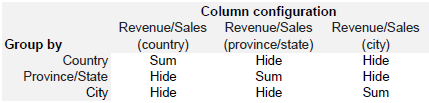A Drill Down Table lets you step through a hierarchy of grouped and summarized data to view varying levels of detail. To do this, the drilldown table must be built such that each column rolls up correctly. In most cases this is straightforward, as numbers are aggregated (summed, averaged, etc) by their grouping, however in certain cases, such as when aggregating ratios, formulas are required to ensure the data aggregates correctly at each level. This article starts with adding a basic drill down to a standard table, then describes how to build an advanced drill down that aggregates ratios.

Important

• All table columns must contain the same number of items and be aligned across rows.
• Only columns formatted as Text or Date/Time can be grouped.
• Only columns formatted asNumber, Currency, Percentage, Duration, Mini Chart: Sparkline or Mini Chart Spark Bar can have arithmetic actions (SUM, AVERAGE, MIN, MAX) applied.
• Custom Results Rows do not automatically recalculate as the user drills down through the levels.
• Filter, Group, Aggregate and Sort actions are applied to the full set of data, before the Drill Down levels are grouped.
• Sort results are accurate at the last ungrouped level.
• Group and Aggregate are typically not needed because Drill Down functionality performs grouping and aggregation.

Standard Table

Each row in this table contains data for every column. In this example, first 6 columns are pulled directly from the datasource and the Revenue/Sales and Revenue/Population columns are straightforward calculations: &Column: Revenue / &Column: Sales and &Column: Revenue / &Column: Population respectively.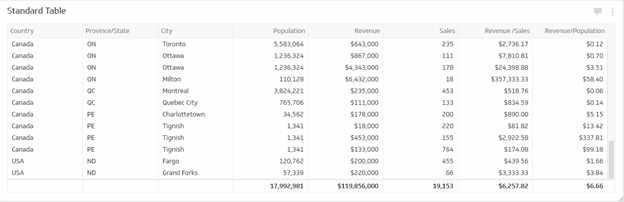Basic Drill Down

The numeric data in this table can be aggregated according to groupings defined by the text fields: Country, Province/State and City. In this Basic Drill Down example, only the Population, Revenue and Sales columns are used.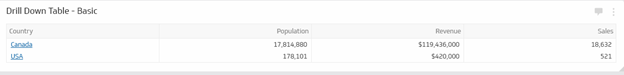Drill Down Configuration

To define the Drill Down path, edit your standard table klip, select the Table in the component hierarchy, then go to the Drill Down tab and select Enable drill down to begin grouping the data.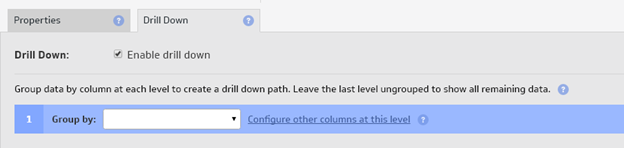The first text column selected to Group by will be the highest level grouping. Click Configure other columns at this level to specify how to handle the data in each of the remaining columns:

• Text and Date/Time column actions are Hide, Count, Count Distinct
• numeric column actions are Hide, Sum, Average, Count, Count Distinct, Min, Max

When a Group by level is selected, the Add Drill Level button is displayed until the final text column is reached. To show all rows, leave the final level ungrouped by setting Group by to blank.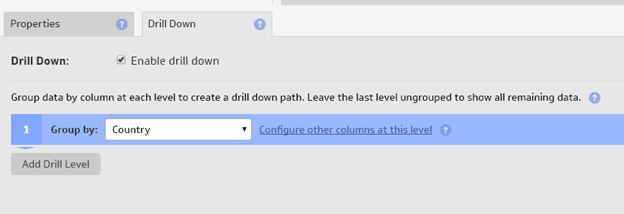NOTE: The maximum number of Drill Levels, including the last ungrouped level, equals the number of text columns in the table. To configure a drill level for every text column and also leave the last level ungrouped to show full details, add an extra hidden column (not hidden data) and set the formula to reference the lowest level grouping (in this example, &Column: City).

Formulas

In the Standard Table, the revenue and sales columns contain unique entries per row which can be aggregated as is. However, the population column gives the total population for the city in each row so must be manipulated in order to aggregate correctly when drilling down through the country, province/state and city levels. To achieve this, the population must be divided by the number of times the city appears in the data using a formula like

population / LOOKUP( city, GROUP(city), COUNTISTINCT(city) )

For example, the Standard Table has 2 rows for Ottawa:

In the Basic Drill Down, the population for Ottawa must be divided by 2 so that it sums correctly at the city level and higher.

Viewing on the Dashboard

Add the Drill Down table klip to a dashboard to view. Click an underlined blue link to drill down to the next level and click Back to return to the previous level.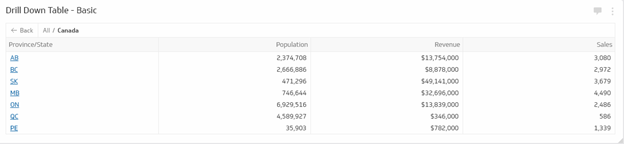In the Advanced Drill Down example, all the numeric columns in the Standard Table (Population, Revenue, Sales, Revenue/Sales, Revenue/Population) will be aggregated according to groupings defined by the text fields: Country, Province/State and City. This example builds on the steps described in the Basic Drill Down section.

To sum ratios such as Revenue/Sales and Revenue/Population requires separately summing the numerator and the denominator, that is:

SUM( &Column: Revenue ) / SUM( &Column: Sales )

SUM( &Column: Revenue ) / SUM( &Column: Population )

A unique formula is required for each level of the Drill Down table since the denominator must be grouped differently for each level (the numerator will be summed as is). For example:

 country province/state city revenue sales revenue/sales Canada ON Toronto 75000 643 116.64 Canada ON Toronto 643000 235 2736.17 Canada ON Ottawa 867000 111 7810.81 Canada ON Ottawa 4343000 178 24398.88

Toronto revenue/sales = (75000 + 643000) / (643 + 235) = 75000/878 + 643000/878 = 817.77

Ottawa revenue/sales = (867000 + 4343000) / (111 + 178) = 867000/289 + 4343000/289 = 18027.68

ON revenue/sales =  (75000 + 643000 + 867000 + 4343000) / (643 + 235 + 111 + 178)

= 75000/1167 +   643000/1167 + 867000/1167 + 4343000/1167 = 5079.69

To do this requires a table column for each level, where: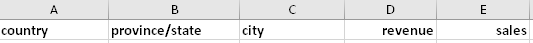• Revenue/Sales (country)

&Column: Revenue /
LOOKUP( &Column: Country,
GROUP(  &Column: Country ),
GROUPBY( &Column: Country, &Column: Sales )

• Revenue/Sales (province/state)

&Column: Revenue /
LOOKUP( CONCAT( &Column: Country, &Column: Province/State ),
GROUP( CONCAT( &Column: Country, &Column: Province/State ) ),
GROUPBY( CONCAT( &Column: Country, &Column: Province/State ), &Column: Sales ) )

• Revenue/Sales (city)

&Column: Revenue /
LOOKUP( CONCAT( &Column: Country, &Column: Province/State, &Column: City ),
GROUP( CONCAT( &Column: Country, &Column: Province/State, Column: City ) ),
GROUPBY( CONCAT( &Column: Country, &Column: Province/State, Column: City ), Column: Sales ) )

On the Drill Down tab, for each level, sum the applicable column and hide the others.